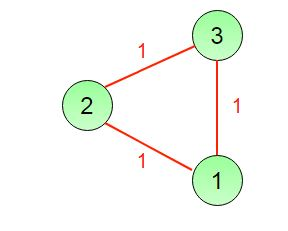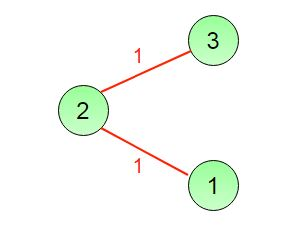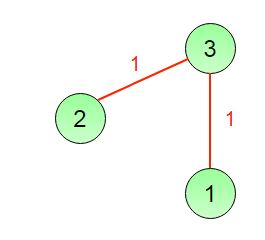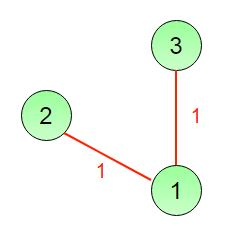Skip to content
Related Articles
Data Structures and Algorithms | Set 22
• Difficulty Level : Medium
• Last Updated : 12 Feb, 2018

Following questions have been asked in GATE CS 2005 exam.

1) A program P reads in 500 integers in the range [0..100] representing the scores of 500 students. It then prints the frequency of each score above 50. What would be the best way for P to store the frequencies?
(a) An array of 50 numbers
(b) An array of 100 numbers
(c) An array of 500 numbers
(d) A dynamically allocated array of 550 numbers

Answer (a)
An array of size 50 looks the best option to store number of students for each score. We need to store frequencies of scores above 50. We can ignore scores below 50 and to index the scores above 50, we can subtract 50 from the score value/

2) An undirected graph G has n nodes. Its adjacency matrix is given by an n × n square matrix whose (i) diagonal elements are 0‘s and (ii) non-diagonal elements are 1‘s. which one of the following is TRUE?

(a) Graph G has no minimum spanning tree (MST)
(b) Graph G has a unique MST of cost n-1
(c) Graph G has multiple distinct MSTs, each of cost n-1
(d) Graph G has multiple spanning trees of different costs

Answer (c)
If all non diagonal elements are 1, then every vertex is connected to every other vertex in the graph with an edge of weight 1. Such a graph has multiple distinct MSTs with cost n-1. See the below example.

The connected graph:Below are three Minimum Spanning trees each of cost 2.0.
Minimum Spanning Tree 1Minimum Spanning Tree 2Minimum Spanning Tree 33) The time complexity of computing the transitive closure of a binary relation on a set of n elements is known to be:
a) O(n)
b) O(nLogn)
c) O(n^(3/2))
d) O(n^3)

Answer (d)
In mathematics, the transitive closure of a binary relation R on a set X is the smallest transitive relation on X that contains R. If the original relation is transitive, the transitive closure will be that same relation; otherwise, the transitive closure will be a different relation.

In computer science the concept of transitive closure can be thought of as constructing a data structure that makes it possible to answer reachability questions. That is, can one get from node a to node other node b in one or more hops? A binary relation tells you only that node a is connected to node b, and that node b is connected to node c, etc. After the transitive closure is constructed in an O(1) operation one may determine that node c is reachable from node a.

Warshall’s algorithm can be used to construct the Transitive closure of directed graphs (). In Warshall’s original formulation of the algorithm, the graph is unweighted and represented by a Boolean adjacency matrix. Then the addition operation is replaced by logical conjunction (AND) and the minimum operation by logical disjunction (OR).

4. A Priority-Queue is implemented as a Max-Heap. Initially, it has 5 elements. The level-order traversal of the heap is given below:
10, 8, 5, 3, 2
Two new elements ”1‘ and ”7‘ are inserted in the heap in that order. The level-order traversal of the heap after the insertion of the elements is:

(a) 10, 8, 7, 5, 3, 2, 1
(b) 10, 8, 7, 2, 3, 1, 5
(c) 10, 8, 7, 1, 2, 3, 5
(d) 10, 8, 7, 3, 2, 1, 5

Answer (D)

Original Max-Heap is:

```        10
/  \
8    5
/ \
3   2
```

After Insertion of 1.

```         10
/    \
8      5
/ \    /
3   2 1
```

After Insertion of 7.

```         10
/   \
8     7
/ \    / \
3   2  1   5
```

Please see GATE Corner for all previous year paper/solutions/explanations, syllabus, important dates, notes, etc.

Please write comments if you find any of the answers/explanations incorrect, or you want to share more information about the topics discussed above.

Attention reader! Don’t stop learning now. Learn all GATE CS concepts with Free Live Classes on our youtube channel.

My Personal Notes arrow_drop_up